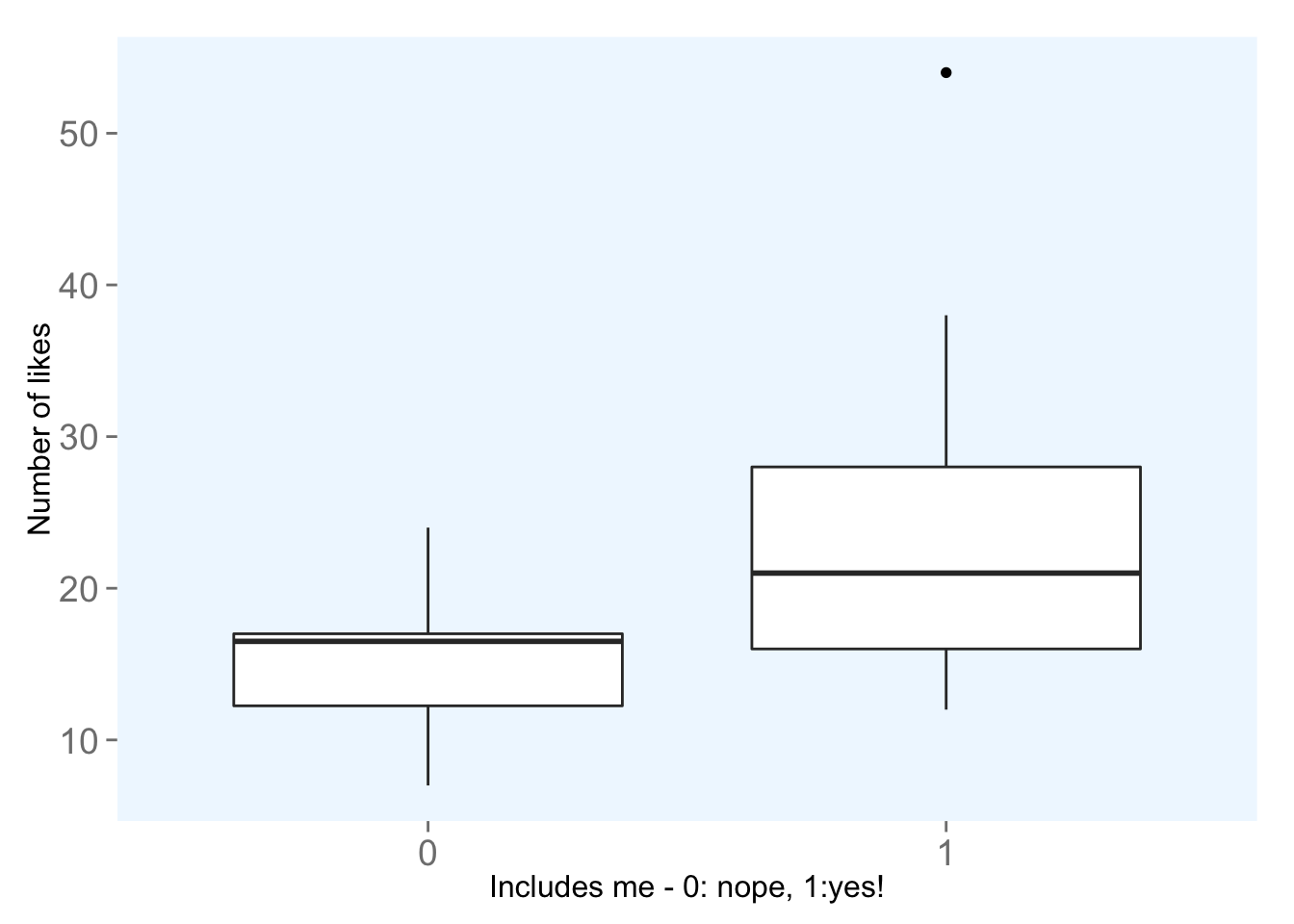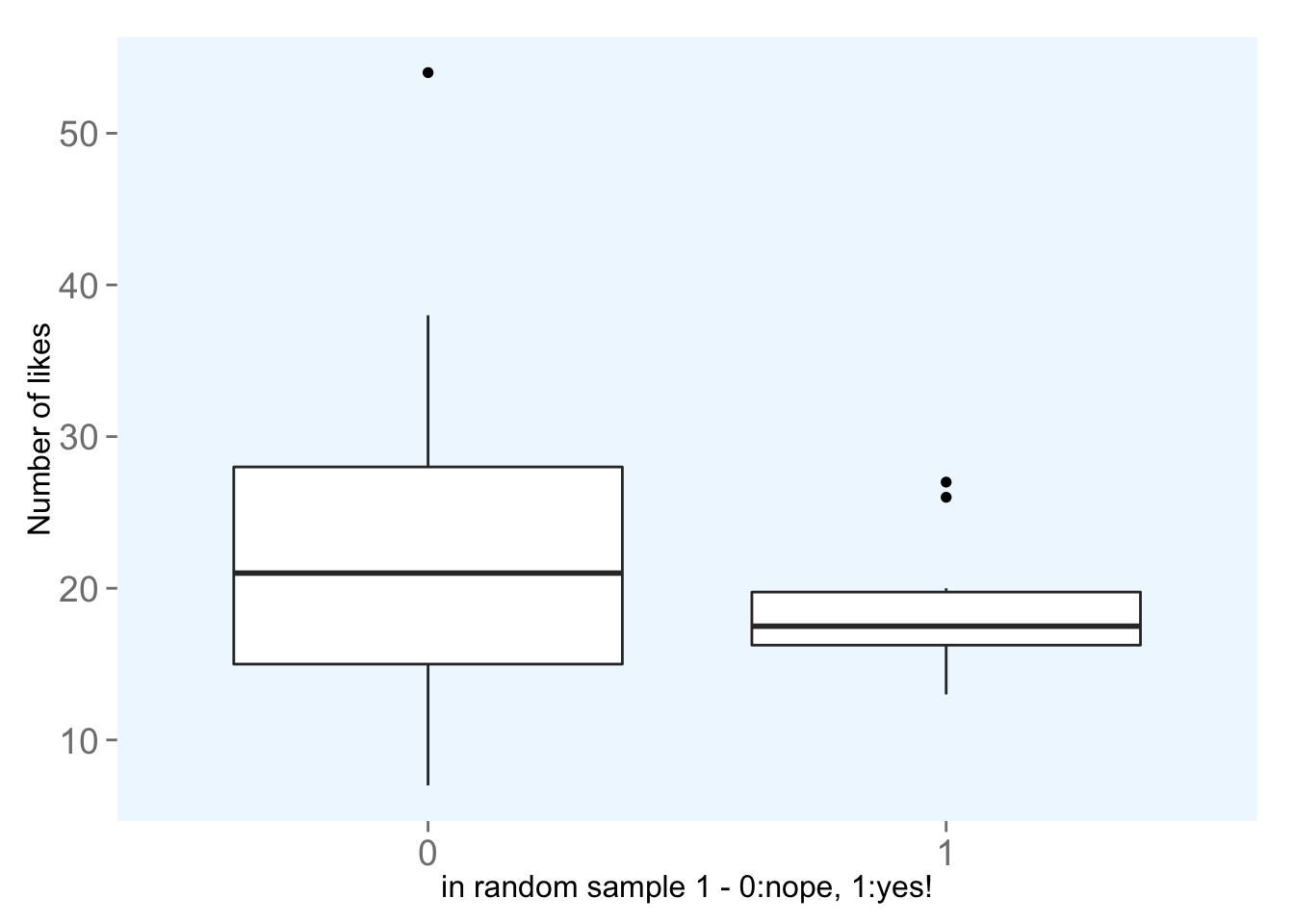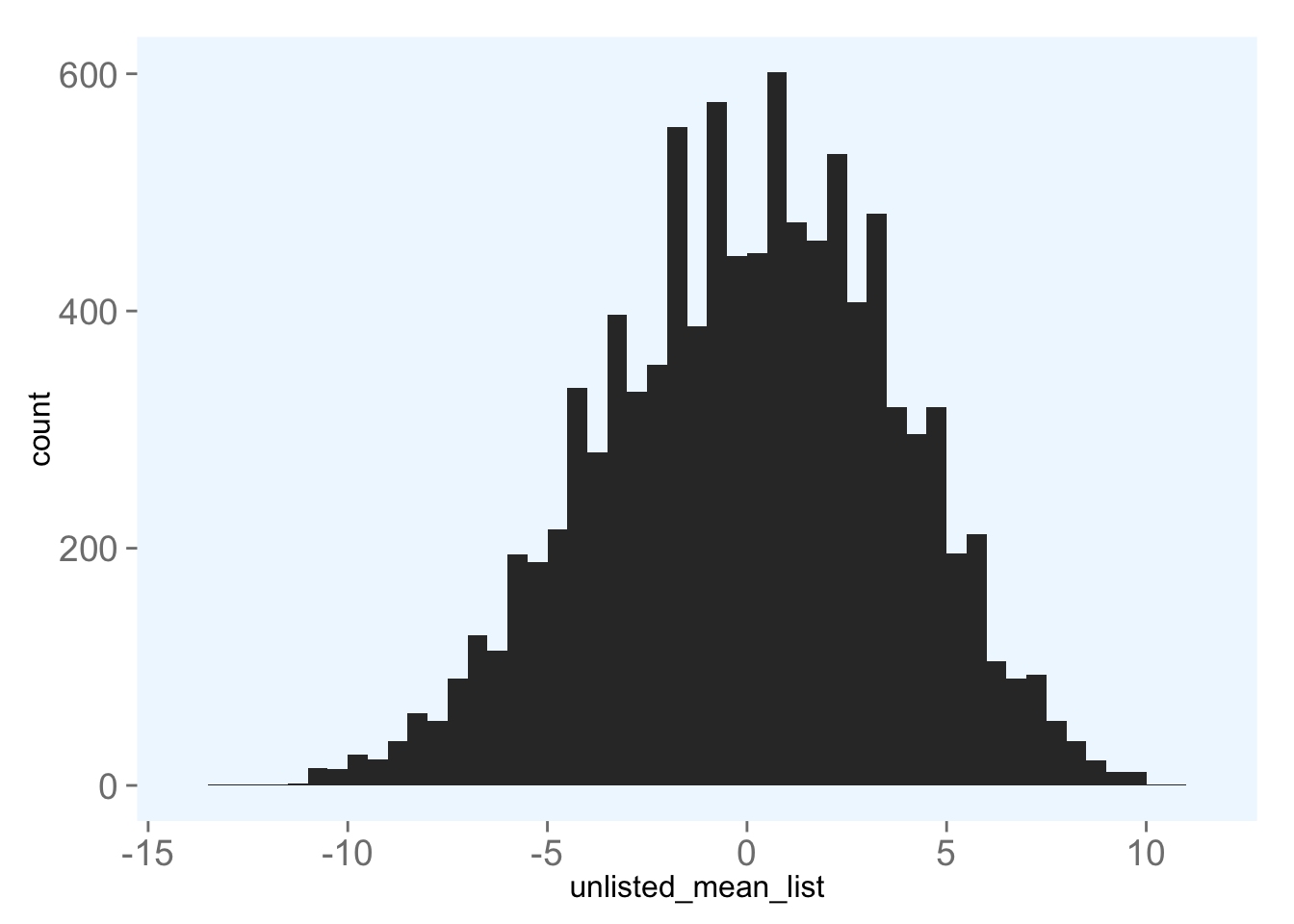## Mann-Whitney U test

I know last time I promised some updates about small n and large p and scraping and this and that and some other nonsense, but I actually got a bit hung up on the Mann-Whitney test that I used last time to determine the differences between the two sample distributions I got for pictures that included me and those that didn’t. As a reminder, those samples look like this:Since the two samples had different variances, I had to use a Mann-Whitney test. I did this a bit blindly, reading one R blogger page and calling it good.

Blind testing is not usually bueno, and since I had never heard of this test before, I decided to do a quick google. As it turns out (according to wikipedia), the main purpose of the Mann-Whitney U test is to reject or not reject the null hypothesis that two samples, we’ll call them X and Y, come from the same umbrella population. Thus, the probability that X > Y is the same as the probability that Y > X.

In our modern age of computing, we can do this (or something pretty similar) with simulations rather than using statistical tests. Since my two samples didn’t have the same number of observations, calculating X - Y, as the Mann-Whitney test in R does isn’t something I can do.

However, we can do one step better by simulating with our actual data and getting an actual probability as our p value - no assumptions required. We can randomly sample from our overall “population” (insta post like data), giving each post an arbitrary assignment to sample X or Y. If we do this sampling, what is the probability that the difference in means between X and Y is greater than or equal to the difference that we saw between the means of the two samples in question: posts that include me and posts that don’t.

## Let’s simulate!

First, let’s remember what our data look like:

##     img_number num_people includes_me num_likes
##  1:          1          1           0         7
##  2:          2          3           1        24
##  3:          3          2           1        35
##  4:          4          2           1        21
##  5:          5          2           1        24
##  6:          6          1           0        12
##  7:          7          0           0         7
##  8:          8          2           1        54
##  9:          9          0           0        18
## 10:         10          1           0        17

Next, we’ll randomly assign our insta data into two different groups. We’ll make sure the sizes of the two groups mirror the sizes of the two groups we care about comparing to: posts with pictures of me (nx) and posts with pictures without me (ny).

nx <- nrow(instaData[includes_me<1 & num_people>0,])
ny <- nrow(instaData[num_people>0]) - nx

Now we can take our random samples and look at the distribution of likes on our two samples!## Now that we’ve done one simulation, let’s do a bunch!

We’ll create a function to do all the dirty work for us:

# create a function to do all the stuff we just did:
picSimulation <- function(data, n, nx, depVar, idVar) {

meanList <- list()

for(i in 1:n){
sampleX <- data[sample(1:nrow(data), nx, replace = F)]
data[['sampleX']] <- ifelse(data[[idVar]] %in% sampleX[[idVar]], 1, 0)

# calculate means of the random samples:
meanX = mean(data[sampleX=='1'][[depVar]])
meanY = mean(data[sampleX=='0'][[depVar]])
diffMeans = meanY - meanX

meanList[i] = diffMeans
}
return(meanList)
}

We can use this function to simulate, 1000 times, and get a distribution of mean differences. That distribution looks like this:# Now let’s have the answer!

Now that we have a distribution of mean differences, let’s figure out the probability that we would get the difference in means we got given that the two came from the same population.

The true difference is 8.795.

Now let’s get the probability (from our distribution!!) that the mean difference would be that large or greater:

nrow(df[unlisted_mean_list > diff])/nrow(df)
##  0.003

Wow! The probability that we would get the mean difference that we did between pics with me and pics without me if there really was no difference between the two is less than one percent - really unlikely! This means that it’s highly likely that the two samples are distinct, that is, they are statistically significantly different from each other!

Feel free to check out the full script on GitHub!